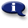# .

## Syllabus Information

Fall 2022
Mar 28,2023Use this page to maintain syllabus information, learning objectives, required materials, and technical requirements for the course.

Syllabus Information
MTH 060D - Beginning Algebra: Part D
Associated Term: Fall 2022
Learning Objectives: Upon successful completion of this course, the student will: 1- Maintain, use, and expand skills and concepts learned in previous mathematics courses. 2- Perform operations with fractions and decimals. 3- Use unit analysis to convert units and solve problems. 4- Interpret information represented numerically and graphically, and recognize linear relationships represented verbally, numerically, graphically, and algebraically. 5- Read and interpret information given in a table or graph. Locate points in a rectangular coordinate system and represent equations in two variables graphically. 6- Identify the horizontal and vertical intercepts of the graph of an equation and interpret them in terms of an application. 7- Identify the slope of a line and interpret it in terms of an application. 8- Use the slope-intercept form of the equation of a line. Make appropriate and efficient use of a scientific calculator. (Note: Students will be expected to demonstrate achievement of some objectives without the use of a calculator.)
Required Materials:
Technical Requirements: